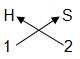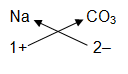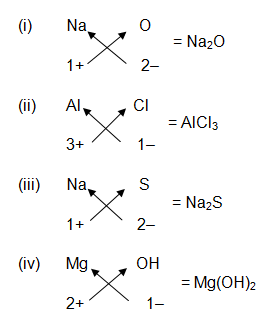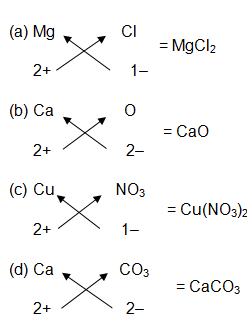# Writing Chemical Formulae

Like in our world, the identity of each person is his/her name, similarly, in chemistry, the first identity of each substance is its chemical formulae. Hence, it is important to write the chemical formula of the compound.

If we know the valencies of elements, then we can work out the formula of their compound by balancing the valencies of the different atoms which occur in the compound. For example, if a compound is made up of hydrogen and sulphur elements, then we should adjust the number of hydrogen atoms and sulphur atoms in such a way that the total valencies of hydrogen atoms become equal to the total valencies of sulphur atoms. This will give us a correct formula of the compound.

Hydrogen sulphide compound is made up of two elements :hydrogen and sulphur. Now, the valency of hydrogen is 1 and that of sulphur is 2. That is:

Valency of one H atom = 1

Valency of one S atom = 2

Now, to balance the valency of 2 of a sulphur atom, we will have to take 2 atoms of hydrogen, because :

Valency of two H atoms = 1 x 2 = 2

This means that 2 atoms of H will combine with 1 atoms of S to form the compound H2S.

There is a criss-cross method to write chemical formula of compounds. The method is given below:

1. We first write the symbols of the elements which form the compound.
2. Below the symbol of each element, we write down its valency.
3. Finally, we cross over the valencies of the combining atoms. That is, with the first atoms we write the valency of second atom (as a subscript); and with the second atom we write the valency of first atom (as subscript). This will give us the required formula.

For example, hydrogen sulphide is made up of hydrogen and sulphur

So first we write its symbols and then valencies. Next, we cross over the valencies to get the final chemical formula.= H2S

### Valency of ions –

In order to write the formulae of ionic compounds, we should know the valencies of positive ions and negative ions which combine to form the ionic compounds. The valency of an ion is equal to the charge on the ion. If an ion has 1 unit charge, its valency is 1 and it is called a monovalent ion. If an ion has 2 units of charge, its valency is 2 and it is called a divalent ion. And if an ion has 3 units of charge, then its valency is 3 and it is called trivalent ion.

A cation has a positive charge, so it has positive valency; an anion has negative charge so, it has negative valency.

Following is the list of monovalent, divalent and trivalent cations and anions.

#### Monovalent cations (cations having a valency of 1+)

Some of the important monovalent cations or monovalent positive ions are:-

1. Hydrogen ion – H+
2. Lithium ion – Li+
3. Sodium ion – Na+
4. Potassium ion – K+
5. Ammonium ion – NH4+
6. Silver ion – Ag+
7. Copper (I) ion [Cuprous ion] – Cu+
8. Mercury (I) ion [Mercurous ion] – Hg+

#### Divalent cations (cations having a valency of 2+)

Some of the important Divalent cations or Divalent positive ions are:-

1. Magnesium ion – Mg2+
2. Calcium ion – Ca2+
3. Zinc ion – Zn2+
4. Iron (II) ion [Ferrous ion] – Fe2+
5. Barium ion – Ba2+
6. Copper (II) ion [Cupric ion] – Cu2+
7. Mercury (II) ion [Mercuric ion] – Hg2+

#### Trivalent cations (cations having a valency of 3+)

Some of the important Trivalent cations or Trivalent positive ions are:-

1. Aluminium ion – Al3+
2. Iron (III) ion [Ferric ion] – Fe3+

#### Monovalent anions (anions having a valency of 1-)

Some of the important Monovalent anions or Monovalent negative ions are:-

1. Hydride ion – H ̶
2. Fluoride ion – F̶
3. Chloride ion – Cl̶
4. Bromide ion – Br̶
5. Iodide ion – I̶
6. Nitrite ion – NO2̶
7. Nitrate ion – NO3̶
8. Hydroxide ion – OH̶
9. Hydrogen carbonate ion [Bicarbonate ion] – HCO3̶

#### Divalent anions (anions having a valency of 2-)

Some of the important Divalent anions or Divalent negative ions are:-

1. Oxide ion – O2 ̶
2. Sulphide ion – S2 ̶
3. Sulphite ion – SO32 ̶
4. Sulphate ion – SO42 ̶
5. Carbonate ion – CO32 ̶

#### Trivalent anions (anions having a valency of 3-)

Some of the important trivalent anions or trivalent negative ions are:-

1. Nitride ion – N3 ̶
2. Phosphide ion – P3 ̶
3. Phosphate ion – PO43 ̶

### Writing formulae of ionic compounds –

To write the formula of ionic compounds, first we write down the name of compound and after that we write the symbols of its ions. As a convention, the cation is written on the left hand side and the anion is written on the left hand side. The number of cations and anions is adjusted in such a way that the total number of positive valencies of cations becomes equal to the total number of negative valencies of anions. The final formula of an ionic compound is written without showing the charges on the ions involved in it.

For example, we have to write the formula of sodium carbonate. Now, sodium carbonate is made up of 2 types of ions: sodium ion, Na+ and carbonate ion, CO32 ̶.First we write down the symbols of sodium ion and carbonate ion. Below the symbol of sodium ion, we write the valency of sodium ion which is 1+. And below the carbonate ion we write the valency of carbonate ion which is 2-.= Na2CO3

### Questions about Chemical Formulae from NCERT textbook –

#### Page 39

Question 1:-

Write down the formulae of :

(i) Sodium oxide
(ii) Aluminium chloride
(iii) Sodium sulphide
(iv) Magnesium hydroxide

Answer:-Question 2:-

Write down the names of compounds represented by the following formulae:

(i) Al2(SO4)3
(ii) CaCl2
(iii) K2SO4
(iv) KNO3
(v) CaCO3

Answer:-

(i) Aluminium sulphate
(ii) Calcium chloride
(iii) Potassium sulphate
(iv) Potassium nitrate
(v) Calcium carbonate

#### Pages 43 and 44

Question 4:-

Write the chemical formulae of the following :

(a) Magnesium chloride
(b) Calcium oxide
(c) Copper nitrate
(d) Aluminium chloride
(e) Calcium carbonate

Answer :-Writing chemical formulae of a compound is very important because the chemical formula gives identity to the compound. The valencies of elements need to be known to write the chemical formulae. The ionic compounds are divided into monovalent, divalent and trivalent ions on the basis of their valencies. We use a criss-cross method to write the chemical formula.